## BERT：理解上下文的語言代表模型¶

• 代表
• representation
• repr.
• repr. 向量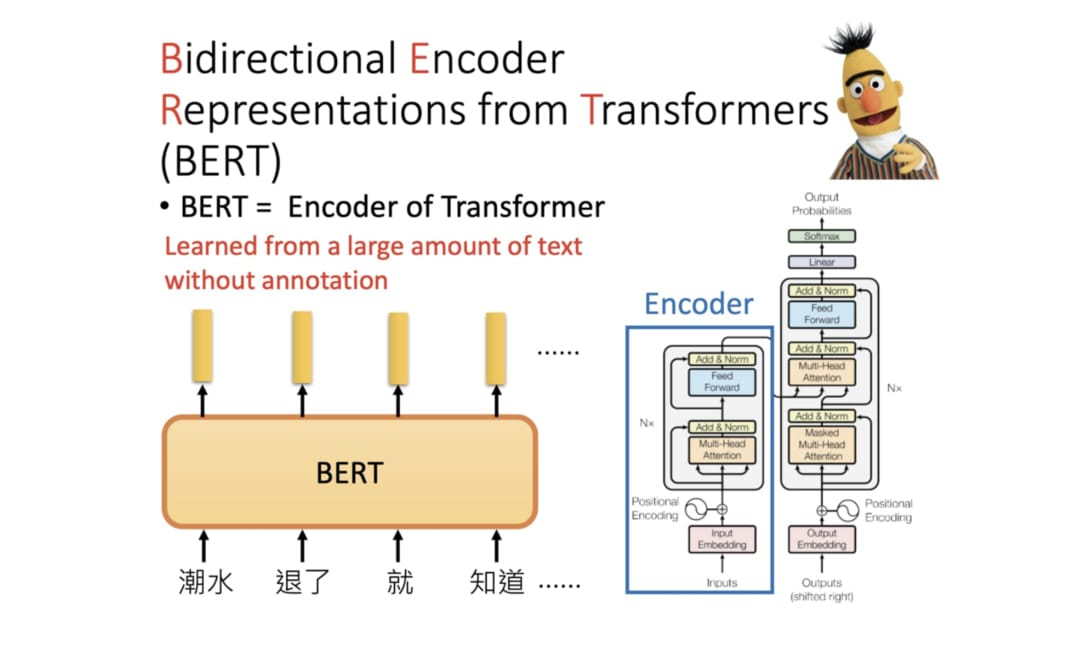BERT 其實就是 Transformer 中的 Encoder，只是有很多層 （圖片來源

BERT 是傳統語言模型的一種變形，而語言模型（Language Model, LM）做的事情就是在給定一些詞彙的前提下， 去估計下一個詞彙出現的機率分佈。在讓 AI 給我們寫點金庸裡的 LSTM 也是一個語言模型 ，只是跟 BERT 差了很多個數量級。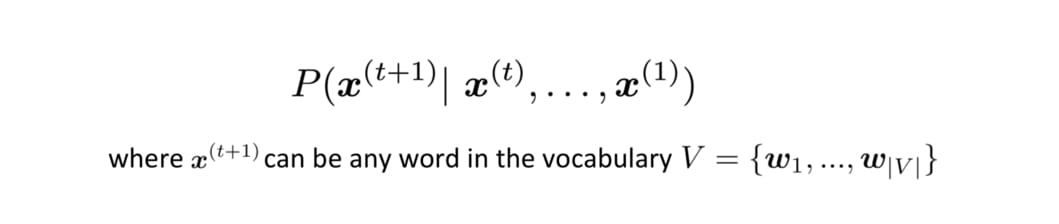• 好處 1：無監督數據無限大。不像 ImageNet 還要找人標注數據，要訓練 LM 的話網路上所有文本都是你潛在的資料集（BERT 預訓練使用的數據集共有 33 個字，其中包含維基百科及 BooksCorpus
• 好處 2：厲害的 LM 能夠學會語法結構、解讀語義甚至指代消解。透過特徵擷取或是 fine-tuning 能更有效率地訓練下游任務並提升其表現
• 好處 3：減少處理不同 NLP 任務所需的 architecture engineering 成本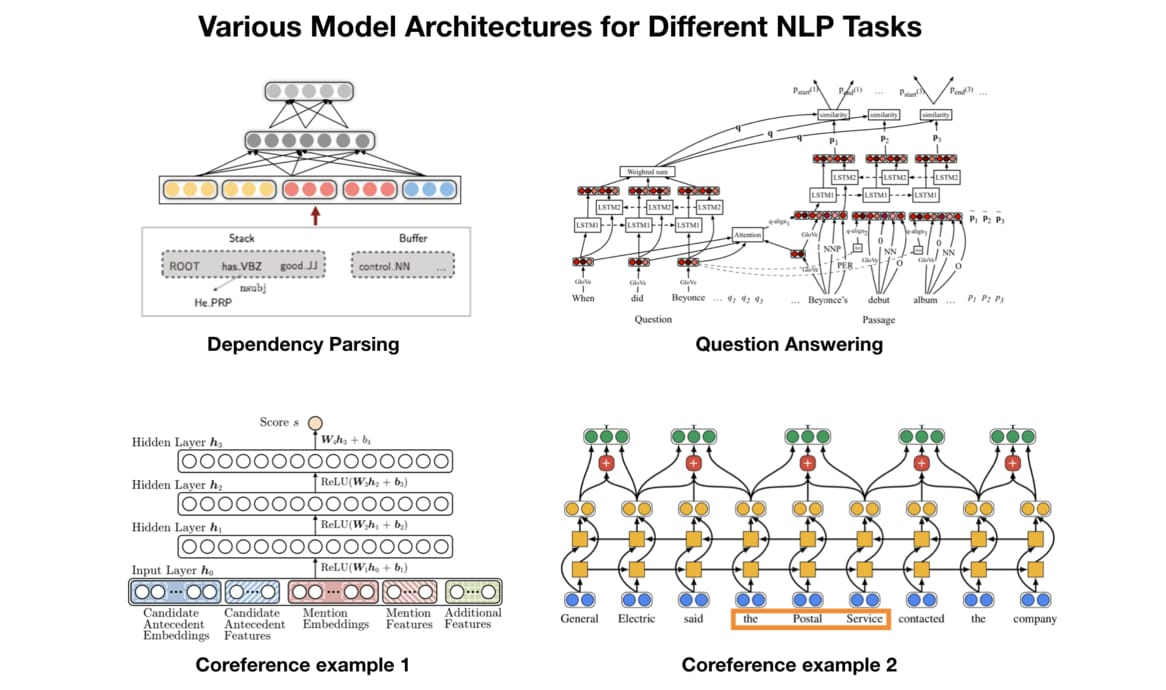• 先以 LM Pretraining 的方式預先訓練出一個對自然語言有一定「理解」的通用模型
• 再將該模型拿來做特徵擷取或是 fine tune 下游的（監督式）任務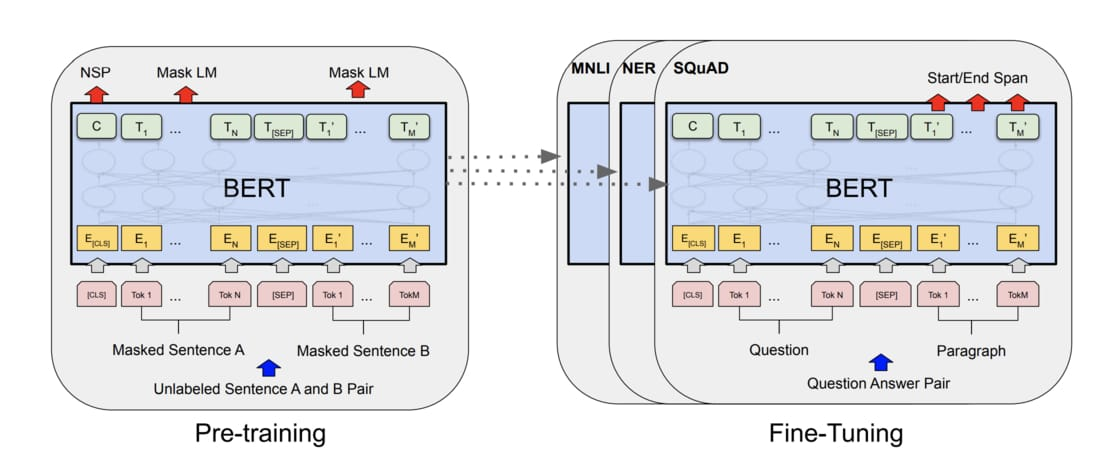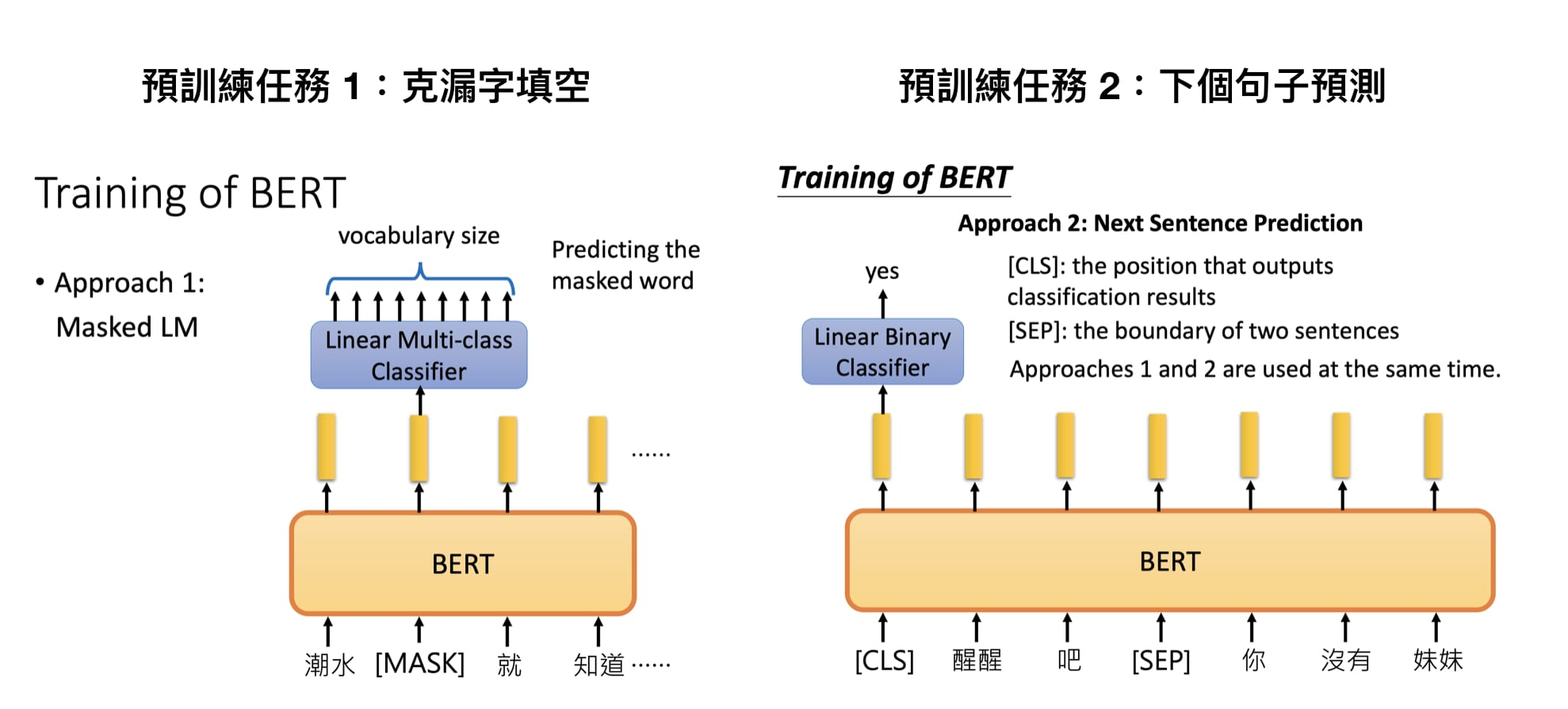BERT 在預訓練時需要完成的兩個任務 （圖片來源

Google 在預訓練 BERT 時讓它同時進行兩個任務：

• 克漏字填空（1953 年被提出的 Cloze task，學術點的說法是 Masked Language Model, MLM）
• 判斷第 2 個句子在原始文本中是否跟第 1 個句子相接（Next Sentence Prediction, NSP）

（2019/10/07 更新：因應 HuggingFace 團隊最近將 GitHub 專案大翻新並更名成 transformers，本文已直接 import 該 repo 並使用新的方法調用 BERT。底下的程式碼將不再使用該團隊在 PyTorch Hub 上 host 的模型。感謝網友 Hsien 提醒）

%%bash
pip install transformers tqdm boto3 requests regex -q


import torch
from transformers import BertTokenizer
from IPython.display import clear_output

PRETRAINED_MODEL_NAME = "bert-base-chinese"  # 指定繁簡中文 BERT-BASE 預訓練模型

# 取得此預訓練模型所使用的 tokenizer
tokenizer = BertTokenizer.from_pretrained(PRETRAINED_MODEL_NAME)

clear_output()
print("PyTorch 版本：", torch.__version__)

PyTorch 版本： 1.4.0


• bert-base-chinese
• bert-base-uncased
• bert-base-cased
• bert-base-german-cased
• bert-base-multilingual-uncased
• bert-base-multilingual-cased
• bert-large-cased
• bert-large-uncased

• 預訓練步驟時用的文本語言
• 有無分大小寫
• 層數的不同
• 預訓練時遮住 wordpieces 或是整個 word

vocab = tokenizer.vocab
print("字典大小：", len(vocab))

字典大小： 21128


import random
random_tokens = random.sample(list(vocab), 10)
random_ids = [vocab[t] for t in random_tokens]

print("{0:20}{1:15}".format("token", "index"))
print("-" * 25)
for t, id in zip(random_tokens, random_ids):
print("{0:15}{1:10}".format(t, id))

token               index
-------------------------
##荘                 18834
##尉                 15259

32gb                11155

##狙                 17376

##诈                 19457

gp                  13228


BERT 使用當初 Google NMT 提出的 WordPiece Tokenization ，將本來的 words 拆成更小粒度的 wordpieces，有效處理不在字典裡頭的詞彙 。中文的話大致上就像是 character-level tokenization，而有 ## 前綴的 tokens 即為 wordpieces。

indices = list(range(647, 657))
some_pairs = [(t, idx) for t, idx in vocab.items() if idx in indices]
for pair in some_pairs:
print(pair)

('ㄅ', 647)
('ㄆ', 648)
('ㄇ', 649)
('ㄉ', 650)
('ㄋ', 651)
('ㄌ', 652)
('ㄍ', 653)
('ㄎ', 654)
('ㄏ', 655)
('ㄒ', 656)


text = "[CLS] 等到潮水 [MASK] 了，就知道誰沒穿褲子。"
tokens = tokenizer.tokenize(text)
ids = tokenizer.convert_tokens_to_ids(tokens)

print(text)
print(tokens[:10], '...')
print(ids[:10], '...')

[CLS] 等到潮水 [MASK] 了，就知道誰沒穿褲子。
['[CLS]', '等', '到', '潮', '水', '[MASK]', '了', '，', '就', '知'] ...
[101, 5023, 1168, 4060, 3717, 103, 749, 8024, 2218, 4761] ...


• [CLS]：在做分類任務時其最後一層的 repr. 會被視為整個輸入序列的 repr.
• [SEP]：有兩個句子的文本會被串接成一個輸入序列，並在兩句之間插入這個 token 以做區隔
• [UNK]：沒出現在 BERT 字典裡頭的字會被這個 token 取代
• [PAD]：zero padding 遮罩，將長度不一的輸入序列補齊方便做 batch 運算
• [MASK]：未知遮罩，僅在預訓練階段會用到

"""

"""
from transformers import BertForMaskedLM

# 除了 tokens 以外我們還需要辨別句子的 segment ids
tokens_tensor = torch.tensor([ids])  # (1, seq_len)
segments_tensors = torch.zeros_like(tokens_tensor)  # (1, seq_len)
clear_output()

# 使用 masked LM 估計 [MASK] 位置所代表的實際 token
outputs = maskedLM_model(tokens_tensor, segments_tensors)
predictions = outputs
# (1, seq_len, num_hidden_units)

# 將 [MASK] 位置的機率分佈取 top k 最有可能的 tokens 出來
k = 3
probs, indices = torch.topk(torch.softmax(predictions[0, masked_index], -1), k)
predicted_tokens = tokenizer.convert_ids_to_tokens(indices.tolist())

# 顯示 top k 可能的字。一般我們就是取 top 1 當作預測值
print("輸入 tokens ：", tokens[:10], '...')
print('-' * 50)
for i, (t, p) in enumerate(zip(predicted_tokens, probs), 1):
print("Top {} ({:2}%)：{}".format(i, int(p.item() * 100), tokens[:10]), '...')

輸入 tokens ： ['[CLS]', '等', '到', '潮', '水', '[MASK]', '了', '，', '就', '知'] ...
--------------------------------------------------
Top 1 (82%)：['[CLS]', '等', '到', '潮', '水', '來', '了', '，', '就', '知'] ...
Top 2 (11%)：['[CLS]', '等', '到', '潮', '水', '濕', '了', '，', '就', '知'] ...
Top 3 ( 2%)：['[CLS]', '等', '到', '潮', '水', '過', '了', '，', '就', '知'] ...


Google 在訓練中文 BERT 鐵定沒看批踢踢，還無法預測出我們最想要的那個 退 字。而最接近的 過 的出現機率只有 2%，但我會說以語言代表模型以及自然語言理解的角度來看這結果已經不差了。BERT 透過關注 潮水 這兩個字，從 2 萬多個 wordpieces 的可能性中選出 來 作為這個情境下 [MASK] token 的預測值 ，也還算說的過去。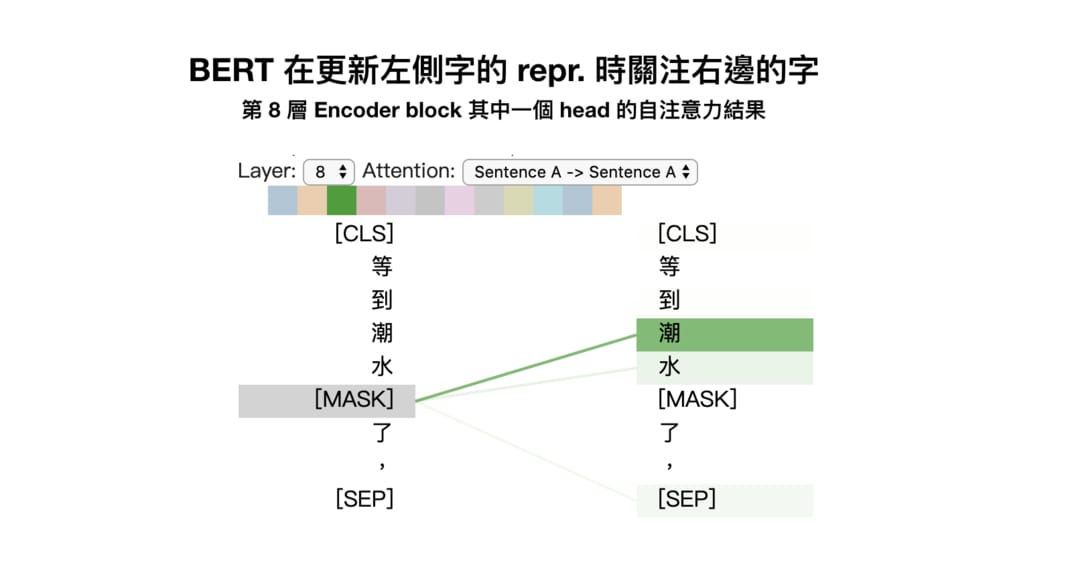Contextual word repr. 讓同 word type 的 word token 在不同語境下有不同的表示方式；而傳統的詞向量無論上下文，都會讓同 type 的 word token 的 repr. 相同。

情境 1：



# 安裝 BertViz
import sys
!test -d bertviz_repo || git clone https://github.com/jessevig/bertviz bertviz_repo
if not 'bertviz_repo' in sys.path:
sys.path += ['bertviz_repo']

# import packages
from transformers import BertTokenizer, BertModel
from bertviz import head_view

# 在 jupyter notebook 裡頭顯示 visualzation 的 helper
def call_html():
import IPython
display(IPython.core.display.HTML('''
<script src="/static/components/requirejs/require.js"></script>
<script>
requirejs.config({
paths: {
base: '/static/base',
"d3": "https://cdnjs.cloudflare.com/ajax/libs/d3/3.5.8/d3.min",
},
});
</script>
'''))

clear_output()


Setup 以後就能非常輕鬆地將 BERT 內部的注意力機制視覺化出來：

# 記得我們是使用中文 BERT
model_version = 'bert-base-chinese'
model = BertModel.from_pretrained(model_version, output_attentions=True)
tokenizer = BertTokenizer.from_pretrained(model_version)

# 情境 1 的句子
sentence_a = "胖虎叫大雄去買漫畫，"
sentence_b = "回來慢了就打他。"

# 得到 tokens 後丟入 BERT 取得 attention
inputs = tokenizer.encode_plus(sentence_a, sentence_b, return_tensors='pt', add_special_tokens=True)
token_type_ids = inputs['token_type_ids']
input_ids = inputs['input_ids']
attention = model(input_ids, token_type_ids=token_type_ids)[-1]
input_id_list = input_ids.tolist() # Batch index 0
tokens = tokenizer.convert_ids_to_tokens(input_id_list)
call_html()

# 交給 BertViz 視覺化

# 注意：執行這段程式碼以後只會顯示下圖左側的結果。
# 為了方便你比較，我把情境 2 的結果也同時附上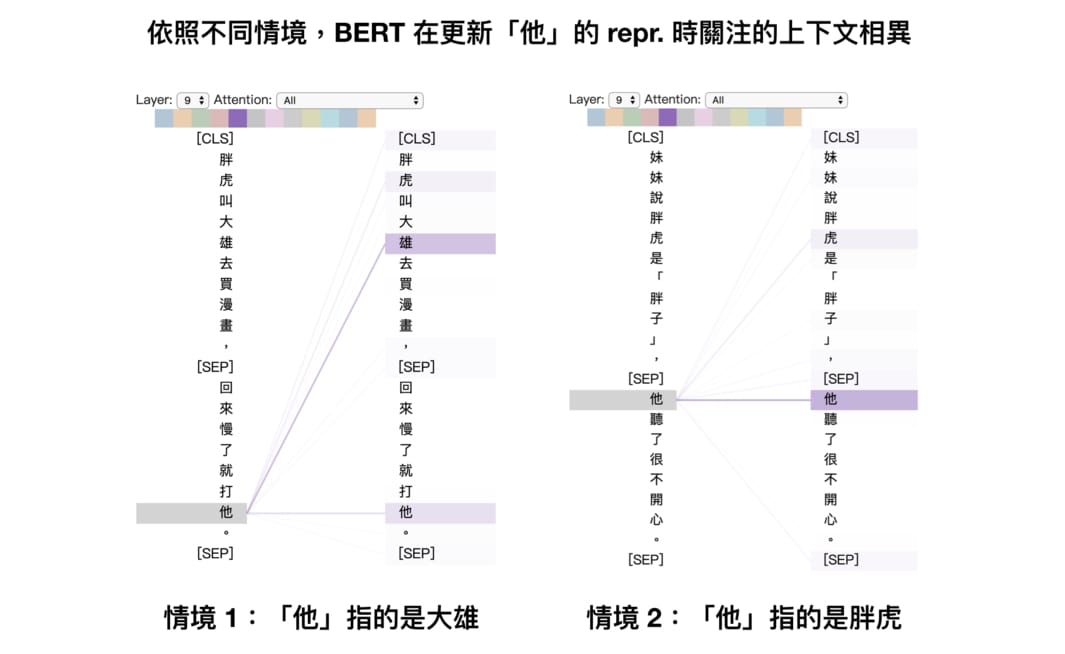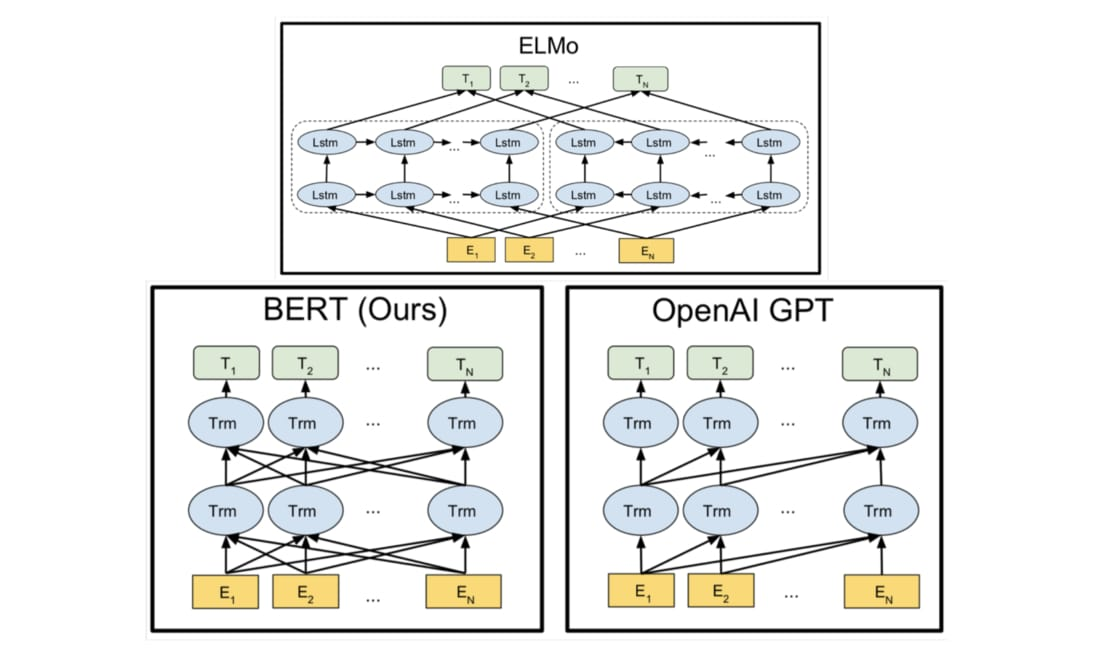ELMo、GPT 以及 BERT 都透過訓練語言模型來獲得 contextual word representation

ELMo 利用獨立訓練的雙向兩層 LSTM 做語言模型並將中間得到的隱狀態向量串接當作每個詞彙的 contextual word repr.；GPT 則是使用 Transformer 的 Decoder 來訓練一個中規中矩，從左到右的單向語言模型。你可以參考我另一篇文章：直觀理解 GPT-2 語言模型並生成金庸武俠小說來深入了解 GPT 與 GPT-2。

BERT 跟它們的差異在於利用 MLM（即克漏字）的概念及 Transformer Encoder 的架構，擺脫以往語言模型只能從單個方向（由左到右或由右到左）估計下個詞彙出現機率的窘境，訓練出一個雙向的語言代表模型。這使得 BERT 輸出的每個 token 的 repr. Tn 都同時蘊含了前後文資訊，真正的雙向 representation。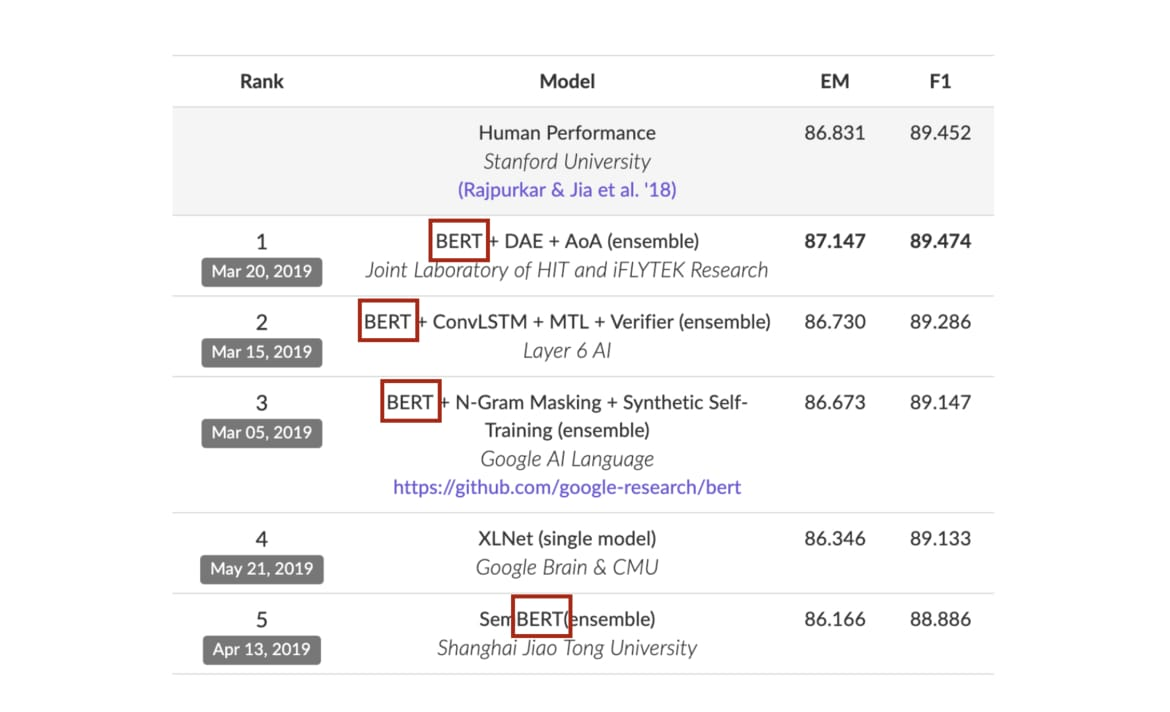SQuAD 2.0 目前排行榜的前 5 名有 4 個有使用 BERT

BERT 是一個強大的語言代表模型，給它一段文本序列，它能回傳一段相同長度且蘊含上下文資訊的 word repr. 序列，對下游的 NLP 任務很有幫助。

## 用 BERT fine tune 下游任務¶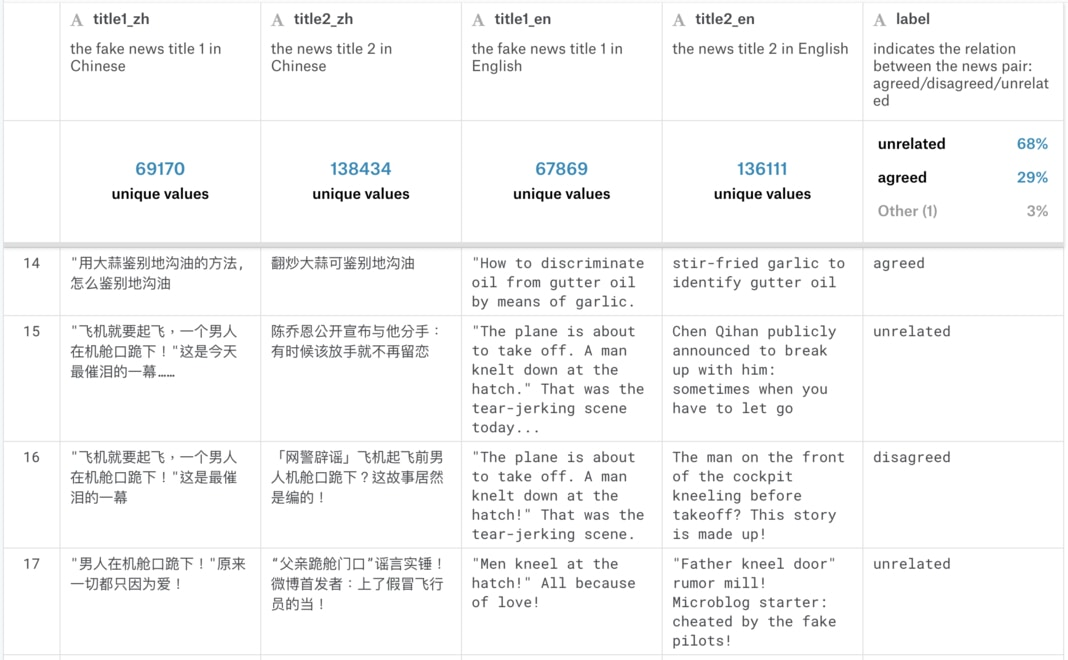fine tune BERT 來解決新的下游任務有 5 個簡單步驟：

• 如何將原始數據轉換成 BERT 相容的輸入格式？
• 如何在 BERT 之上建立 layer(s) 以符合下游任務需求？

### 1. 準備原始文本數據¶

import glob
glob.glob("*.csv.zip")

['train.csv.zip', 'test.csv.zip']

"""

"""
import os
import pandas as pd

# 解壓縮從 Kaggle 競賽下載的訓練壓縮檔案
os.system("unzip train.csv.zip")

# 簡單的數據清理，去除空白標題的 examples
empty_title = ((df_train['title2_zh'].isnull()) \
| (df_train['title1_zh'].isnull()) \
| (df_train['title2_zh'] == '') \
| (df_train['title2_zh'] == '0'))
df_train = df_train[~empty_title]

# 剔除過長的樣本以避免 BERT 無法將整個輸入序列放入記憶體不多的 GPU
MAX_LENGTH = 30
df_train = df_train[~(df_train.title1_zh.apply(lambda x : len(x)) > MAX_LENGTH)]
df_train = df_train[~(df_train.title2_zh.apply(lambda x : len(x)) > MAX_LENGTH)]

# 只用 1% 訓練數據看看 BERT 對少量標註數據有多少幫助
SAMPLE_FRAC = 0.01
df_train = df_train.sample(frac=SAMPLE_FRAC, random_state=9527)

# 去除不必要的欄位並重新命名兩標題的欄位名
df_train = df_train.reset_index()
df_train = df_train.loc[:, ['title1_zh', 'title2_zh', 'label']]
df_train.columns = ['text_a', 'text_b', 'label']

# idempotence, 將處理結果另存成 tsv 供 PyTorch 使用
df_train.to_csv("train.tsv", sep="\t", index=False)

print("訓練樣本數：", len(df_train))df_train.label.value_counts() / len(df_train)

unrelated    0.679338
agreed       0.294317
disagreed    0.026346
Name: label, dtype: float64

os.system("unzip test.csv.zip")
df_test = df_test.loc[:, ["title1_zh", "title2_zh", "id"]]
df_test.columns = ["text_a", "text_b", "Id"]
df_test.to_csv("test.tsv", sep="\t", index=False)

print("預測樣本數：", len(df_test))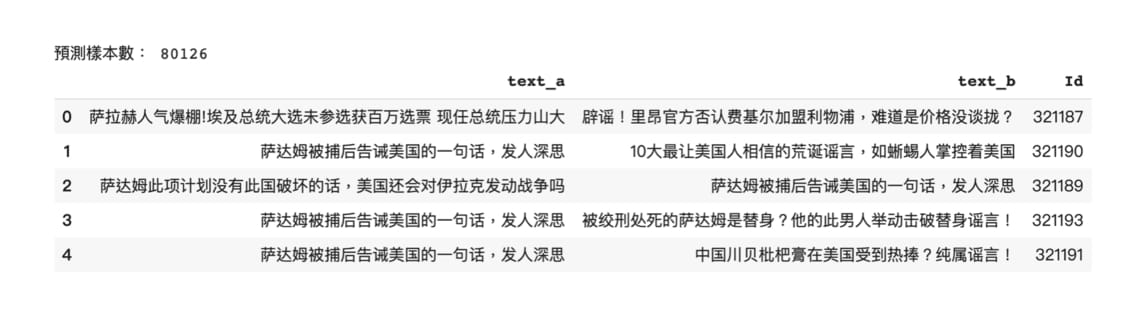ratio = len(df_test) / len(df_train)
print("測試集樣本數 / 訓練集樣本數 = {:.1f} 倍".format(ratio))

測試集樣本數 / 訓練集樣本數 = 30.2 倍


### 2. 將原始文本轉換成 BERT 相容的輸入格式¶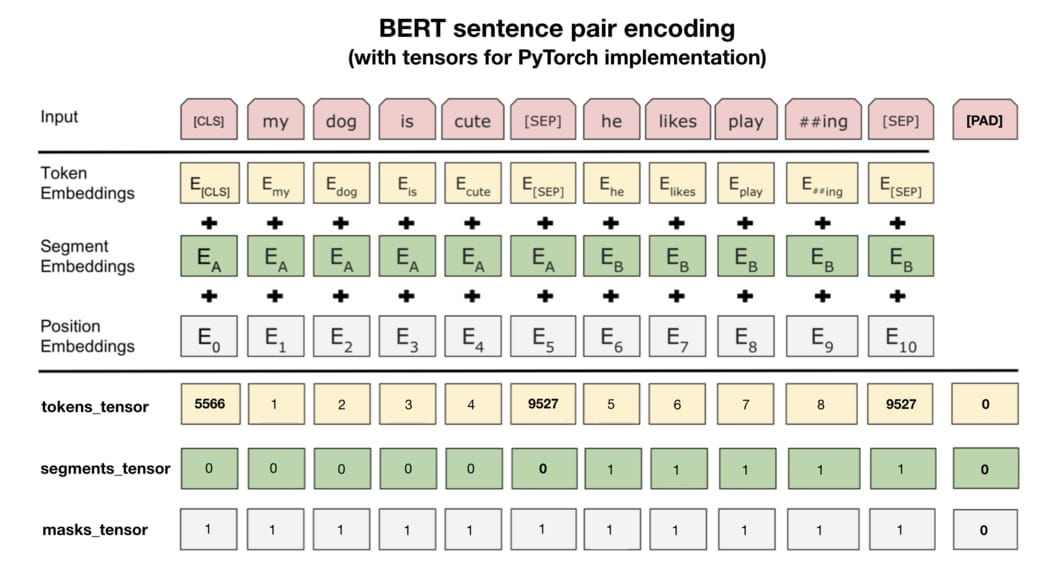• tokens_tensor：代表識別每個 token 的索引值，用 tokenizer 轉換即可
• segments_tensor：用來識別句子界限。第一句為 0，第二句則為 1。另外注意句子間的 [SEP] 為 0
• masks_tensor：用來界定自注意力機制範圍。1 讓 BERT 關注該位置，0 則代表是 padding 不需關注

"""

- tokens_tensor：兩個句子合併後的索引序列，包含 [CLS] 與 [SEP]
- segments_tensor：可以用來識別兩個句子界限的 binary tensor
- label_tensor：將分類標籤轉換成類別索引的 tensor, 如果是測試集則回傳 None
"""
from torch.utils.data import Dataset

class FakeNewsDataset(Dataset):
# 讀取前處理後的 tsv 檔並初始化一些參數
def __init__(self, mode, tokenizer):
assert mode in ["train", "test"]  # 一般訓練你會需要 dev set
self.mode = mode
# 大數據你會需要用 iterator=True
self.df = pd.read_csv(mode + ".tsv", sep="\t").fillna("")
self.len = len(self.df)
self.label_map = {'agreed': 0, 'disagreed': 1, 'unrelated': 2}
self.tokenizer = tokenizer  # 我們將使用 BERT tokenizer

# 定義回傳一筆訓練 / 測試數據的函式
def __getitem__(self, idx):
if self.mode == "test":
text_a, text_b = self.df.iloc[idx, :2].values
label_tensor = None
else:
text_a, text_b, label = self.df.iloc[idx, :].values
# 將 label 文字也轉換成索引方便轉換成 tensor
label_id = self.label_map[label]
label_tensor = torch.tensor(label_id)

# 建立第一個句子的 BERT tokens 並加入分隔符號 [SEP]
word_pieces = ["[CLS]"]
tokens_a = self.tokenizer.tokenize(text_a)
word_pieces += tokens_a + ["[SEP]"]
len_a = len(word_pieces)

# 第二個句子的 BERT tokens
tokens_b = self.tokenizer.tokenize(text_b)
word_pieces += tokens_b + ["[SEP]"]
len_b = len(word_pieces) - len_a

# 將整個 token 序列轉換成索引序列
ids = self.tokenizer.convert_tokens_to_ids(word_pieces)
tokens_tensor = torch.tensor(ids)

# 將第一句包含 [SEP] 的 token 位置設為 0，其他為 1 表示第二句
segments_tensor = torch.tensor( * len_a +  * len_b,
dtype=torch.long)

return (tokens_tensor, segments_tensor, label_tensor)

def __len__(self):
return self.len

# 初始化一個專門讀取訓練樣本的 Dataset，使用中文 BERT 斷詞
trainset = FakeNewsDataset("train", tokenizer=tokenizer)


# 選擇第一個樣本
sample_idx = 0

# 將原始文本拿出做比較
text_a, text_b, label = trainset.df.iloc[sample_idx].values

# 利用剛剛建立的 Dataset 取出轉換後的 id tensors
tokens_tensor, segments_tensor, label_tensor = trainset[sample_idx]

# 將 tokens_tensor 還原成文本
tokens = tokenizer.convert_ids_to_tokens(tokens_tensor.tolist())
combined_text = "".join(tokens)

# 渲染前後差異，毫無反應就是個 print。可以直接看輸出結果
print(f"""[原始文本]

--------------------

[Dataset 回傳的 tensors]
tokens_tensor  ：{tokens_tensor}

segments_tensor：{segments_tensor}

label_tensor   ：{label_tensor}

--------------------

[還原 tokens_tensors]
{combined_text}
""")

[原始文本]

--------------------

[Dataset 回傳的 tensors]
tokens_tensor  ：tensor([ 101, 5722, 3300, 3301, 6206, 5310, 2042,  749, 8024,  852, 5381, 1351,
6230, 2533,  800, 6820, 3221, 1469, 3360, 2552, 1963, 3683, 6772, 1394,
6844,  102, 1962, 7318, 6057, 5310, 2042, 5314,  679, 2042, 3184, 4638,
4912, 2269, 2803, 5709, 4413, 8024,  948, 7450, 4638, 4912, 2269, 2957,
3717, 7027, 5010, 1526, 5722, 3300, 3301, 8013,  102])

segments_tensor：tensor([0, 0, 0, 0, 0, 0, 0, 0, 0, 0, 0, 0, 0, 0, 0, 0, 0, 0, 0, 0, 0, 0, 0, 0,
0, 0, 1, 1, 1, 1, 1, 1, 1, 1, 1, 1, 1, 1, 1, 1, 1, 1, 1, 1, 1, 1, 1, 1,
1, 1, 1, 1, 1, 1, 1, 1, 1])

label_tensor   ：2

--------------------

[還原 tokens_tensors]
[CLS]苏有朋要结婚了，但网友觉得他还是和林心如比较合适[SEP]好闺蜜结婚给不婚族的秦岚扔花球，倒霉的秦岚掉水里笑哭苏有朋！[SEP]



!pip install pysnooper -q
import pysnooper

class FakeNewsDataset(Dataset):
...
@pysnooper.snoop()  # 加入以了解所有轉換過程
def __getitem__(self, idx):
...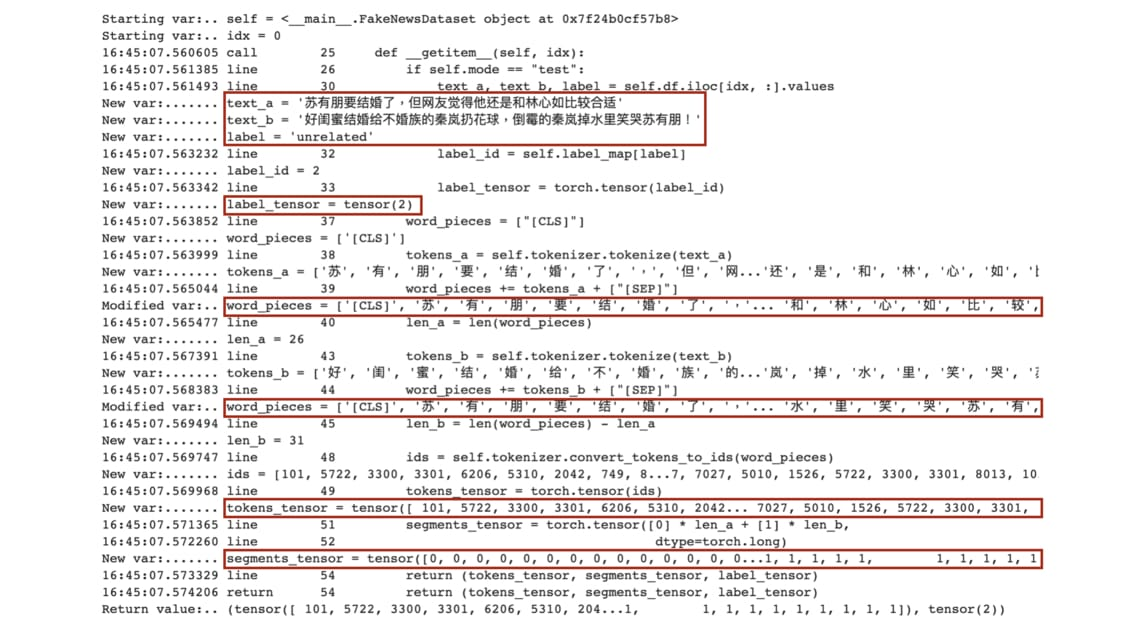"""

- tokens_tensors  : (batch_size, max_seq_len_in_batch)
- segments_tensors: (batch_size, max_seq_len_in_batch)
- masks_tensors   : (batch_size, max_seq_len_in_batch)
- label_ids       : (batch_size)
"""

from torch.utils.data import DataLoader
from torch.nn.utils.rnn import pad_sequence

# 這個函式的輸入 samples 是一個 list，裡頭的每個 element 都是
# 剛剛定義的 FakeNewsDataset 回傳的一個樣本，每個樣本都包含 3 tensors：
# - tokens_tensor
# - segments_tensor
# - label_tensor
# 它會對前兩個 tensors 作 zero padding，並產生前面說明過的 masks_tensors
def create_mini_batch(samples):
tokens_tensors = [s for s in samples]
segments_tensors = [s for s in samples]

# 測試集有 labels
if samples is not None:
label_ids = torch.stack([s for s in samples])
else:
label_ids = None

# zero pad 到同一序列長度
batch_first=True)
batch_first=True)

# attention masks，將 tokens_tensors 裡頭不為 zero padding
# 的位置設為 1 讓 BERT 只關注這些位置的 tokens
dtype=torch.long)
tokens_tensors != 0, 1)

# 初始化一個每次回傳 64 個訓練樣本的 DataLoader
# 利用 collate_fn 將 list of samples 合併成一個 mini-batch 是關鍵
BATCH_SIZE = 64
collate_fn=create_mini_batch)


data = next(iter(trainloader))

tokens_tensors, segments_tensors, \
masks_tensors, label_ids = data

print(f"""
tokens_tensors.shape   = {tokens_tensors.shape}
{tokens_tensors}
------------------------
segments_tensors.shape = {segments_tensors.shape}
{segments_tensors}
------------------------
------------------------
label_ids.shape        = {label_ids.shape}
{label_ids}
""")

tokens_tensors.shape   = torch.Size([64, 63])
tensor([[ 101, 5722, 3300,  ...,    0,    0,    0],
[ 101, 4255, 3160,  ..., 8013,  102,    0],
[ 101,  711, 2506,  ..., 8013,  102,    0],
...,
[ 101,  671, 2157,  ...,    0,    0,    0],
[ 101, 1380,  677,  ...,    0,    0,    0],
[ 101, 2458, 1853,  ...,    0,    0,    0]])
------------------------
segments_tensors.shape = torch.Size([64, 63])
tensor([[0, 0, 0,  ..., 0, 0, 0],
[0, 0, 0,  ..., 1, 1, 0],
[0, 0, 0,  ..., 1, 1, 0],
...,
[0, 0, 0,  ..., 0, 0, 0],
[0, 0, 0,  ..., 0, 0, 0],
[0, 0, 0,  ..., 0, 0, 0]])
------------------------
masks_tensors.shape    = torch.Size([64, 63])
tensor([[1, 1, 1,  ..., 0, 0, 0],
[1, 1, 1,  ..., 1, 1, 0],
[1, 1, 1,  ..., 1, 1, 0],
...,
[1, 1, 1,  ..., 0, 0, 0],
[1, 1, 1,  ..., 0, 0, 0],
[1, 1, 1,  ..., 0, 0, 0]])
------------------------
label_ids.shape        = torch.Size()
tensor([2, 0, 2, 2, 1, 2, 0, 2, 2, 0, 0, 2, 2, 2, 2, 2, 2, 2, 2, 2, 2, 0, 2, 2,
2, 2, 2, 2, 0, 2, 2, 2, 2, 1, 2, 0, 2, 0, 2, 2, 2, 2, 2, 0, 2, 2, 2, 0,
0, 2, 0, 2, 2, 0, 2, 2, 0, 2, 2, 0, 0, 2, 0, 0])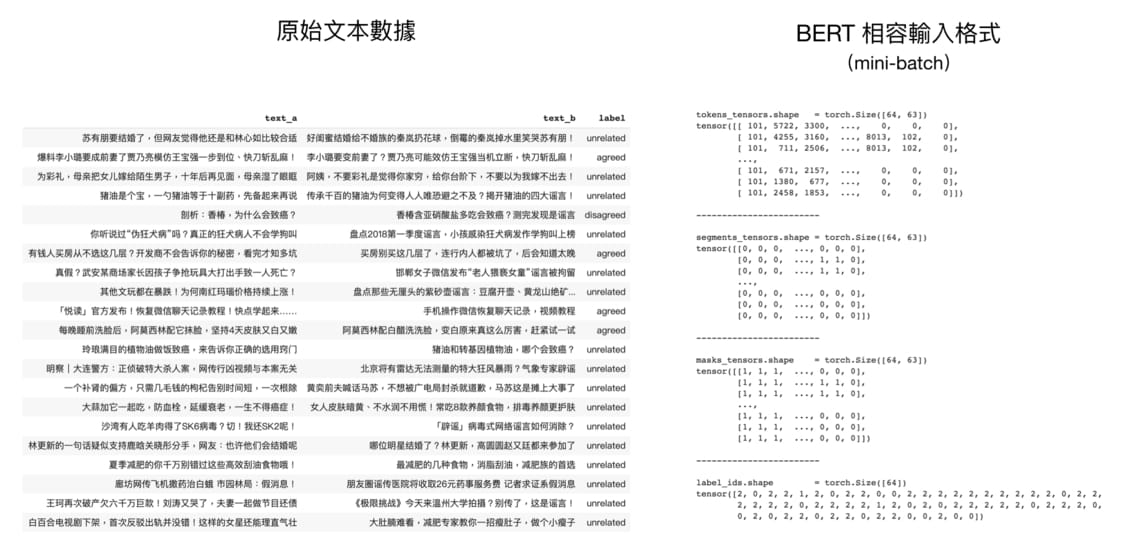### 3. 在 BERT 之上加入新 layer 成下游任務模型¶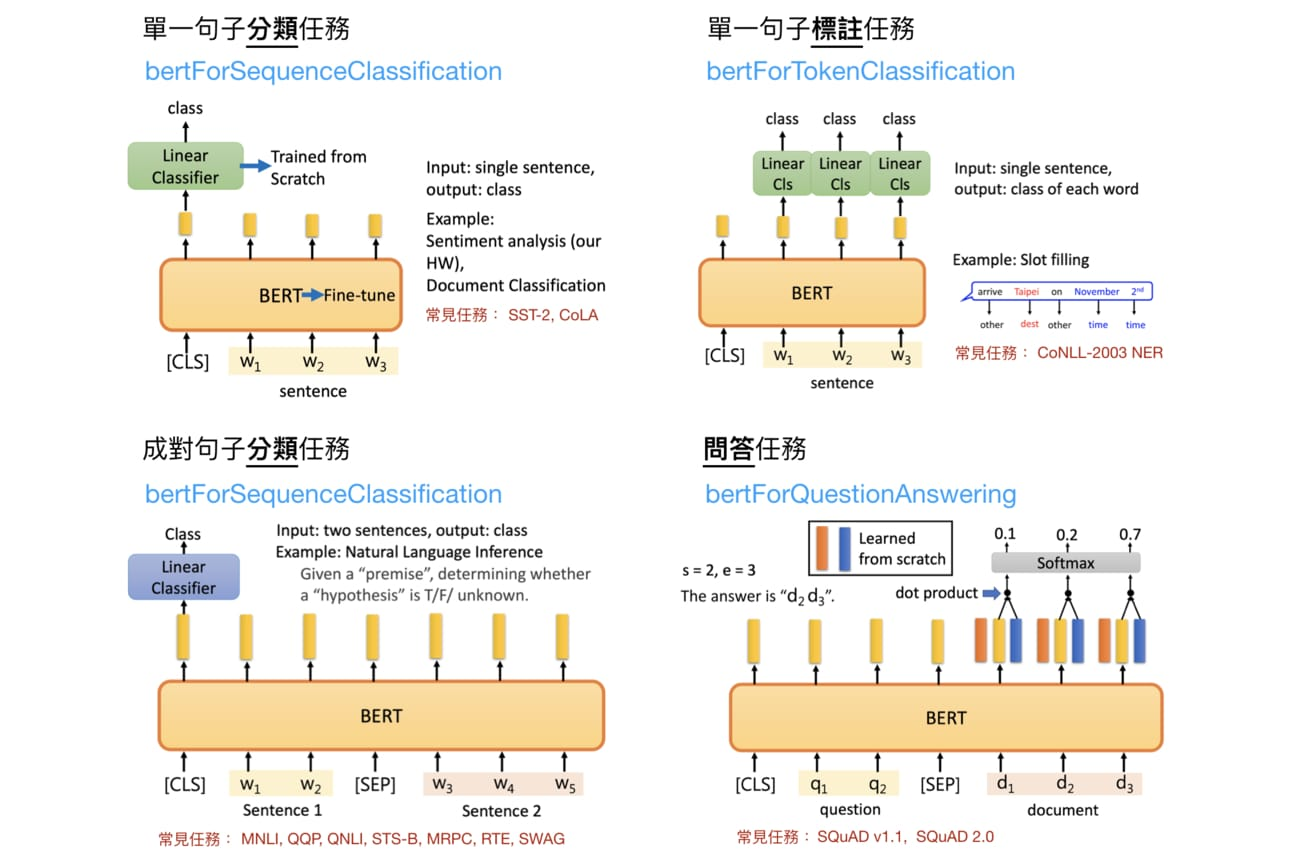# 載入一個可以做中文多分類任務的模型，n_class = 3
from transformers import BertForSequenceClassification

PRETRAINED_MODEL_NAME = "bert-base-chinese"
NUM_LABELS = 3

model = BertForSequenceClassification.from_pretrained(
PRETRAINED_MODEL_NAME, num_labels=NUM_LABELS)

clear_output()

# high-level 顯示此模型裡的 modules
print("""
name            module
----------------------""")
for name, module in model.named_children():
if name == "bert":
for n, _ in module.named_children():
print(f"{name}:{n}")
else:
print("{:15} {}".format(name, module))

name            module
----------------------
bert:embeddings
bert:encoder
bert:pooler
dropout         Dropout(p=0.1, inplace=False)
classifier      Linear(in_features=768, out_features=3, bias=True)


• BERT 處理各種 embeddings 的模組
• 神經機器翻譯就已經看過的 Transformer Encoder
• 一個 pool [CLS] token 在所有層的 repr. 的 BertPooler
• Dropout 層
• 回傳 3 個類別 logits 的線性分類器 classifier

classifer 就只是將從 BERT 那邊拿到的 [CLS] token 的 repr. 做一個線性轉換而已，非常簡單。我也將我們實際使用的分類模型 BertForSequenceClassification 實作簡化一下供你參考：

class BertForSequenceClassification(BertPreTrainedModel):
def __init__(self, config, num_labels=2, ...):
super(BertForSequenceClassification, self).__init__(config)
self.num_labels = num_labels
self.bert = BertModel(config, ...)  # 載入預訓練 BERT
self.dropout = nn.Dropout(config.hidden_dropout_prob)
# 簡單 linear 層
self.classifier = nn.Linear(config.hidden_size, num_labels)
...

def forward(self, input_ids, token_type_ids=None, attention_mask=None, labels=None, ...):
# BERT 輸入就是 tokens, segments, masks
outputs = self.bert(input_ids, token_type_ids, attention_mask, ...)
...
pooled_output = self.dropout(pooled_output)
# 線性分類器將 dropout 後的 BERT repr. 轉成類別 logits
logits = self.classifier(pooled_output)

# 輸入有 labels 的話直接計算 Cross Entropy 回傳，方便！
if labels is not None:
loss_fct = CrossEntropyLoss()
loss = loss_fct(logits.view(-1, self.num_labels), labels.view(-1))
return loss
# 有要求回傳注意矩陣的話回傳
elif self.output_attentions:
return all_attentions, logits
# 回傳各類別的 logits
return logits


model.config

BertConfig {
"architectures": [
],
"attention_probs_dropout_prob": 0.1,
"bos_token_id": 0,
"directionality": "bidi",
"do_sample": false,
"eos_token_ids": 0,
"hidden_act": "gelu",
"hidden_dropout_prob": 0.1,
"hidden_size": 768,
"id2label": {
"0": "LABEL_0",
"1": "LABEL_1"
},
"initializer_range": 0.02,
"intermediate_size": 3072,
"is_decoder": false,
"label2id": {
"LABEL_0": 0,
"LABEL_1": 1
},
"layer_norm_eps": 1e-12,
"length_penalty": 1.0,
"max_length": 20,
"max_position_embeddings": 512,
"model_type": "bert",
"num_beams": 1,
"num_hidden_layers": 12,
"num_labels": 3,
"num_return_sequences": 1,
"output_attentions": false,
"output_hidden_states": false,
"output_past": true,
"pooler_fc_size": 768,
"pooler_num_fc_layers": 3,
"pooler_type": "first_token_transform",
"repetition_penalty": 1.0,
"temperature": 1.0,
"top_k": 50,
"top_p": 1.0,
"torchscript": false,
"type_vocab_size": 2,
"use_bfloat16": false,
"vocab_size": 21128
}

Dropout、LayerNorm、全連接層數以及 mutli-head attentions 的 num_attention_heads 等超參數我們也都已經在之前的 Transformer 文章看過了，這邊就不再贅述。

• 基本款：
• bertModel
• bertTokenizer
• 預訓練階段
• bertForNextSentencePrediction
• bertForPreTraining
• Fine-tuning 階段
• bertForSequenceClassification
• bertForTokenClassification
• bertForMultipleChoice

"""

2019/11/22 更新：在將 tokens、segments_tensors 等 tensors

"""

def get_predictions(model, dataloader, compute_acc=False):
predictions = None
correct = 0
total = 0

# 遍巡整個資料集
for data in dataloader:
# 將所有 tensors 移到 GPU 上
if next(model.parameters()).is_cuda:
data = [t.to("cuda:0") for t in data if t is not None]

# 別忘記前 3 個 tensors 分別為 tokens, segments 以及 masks
# 且強烈建議在將這些 tensors 丟入 model 時指定對應的參數名稱
tokens_tensors, segments_tensors, masks_tensors = data[:3]
outputs = model(input_ids=tokens_tensors,
token_type_ids=segments_tensors,

logits = outputs
_, pred = torch.max(logits.data, 1)

# 用來計算訓練集的分類準確率
if compute_acc:
labels = data
total += labels.size(0)
correct += (pred == labels).sum().item()

# 將當前 batch 記錄下來
if predictions is None:
predictions = pred
else:
predictions = torch.cat((predictions, pred))

if compute_acc:
acc = correct / total
return predictions, acc
return predictions

# 讓模型跑在 GPU 上並取得訓練集的分類準確率
device = torch.device("cuda:0" if torch.cuda.is_available() else "cpu")
print("device:", device)
model = model.to(device)
_, acc = get_predictions(model, trainloader, compute_acc=True)
print("classification acc:", acc)

device: cuda:0
classification acc: 0.03387278885961611


def get_learnable_params(module):
return [p for p in module.parameters() if p.requires_grad]

model_params = get_learnable_params(model)
clf_params = get_learnable_params(model.classifier)

print(f"""

""")

整個分類模型的參數量：102269955



### 4. 訓練該下游任務模型¶

%%time

# 訓練模式
model.train()

# 使用 Adam Optim 更新整個分類模型的參數
optimizer = torch.optim.Adam(model.parameters(), lr=1e-5)

EPOCHS = 6  # 幸運數字
for epoch in range(EPOCHS):

running_loss = 0.0
for data in trainloader:

tokens_tensors, segments_tensors, \
masks_tensors, labels = [t.to(device) for t in data]

# 將參數梯度歸零

# forward pass
outputs = model(input_ids=tokens_tensors,
token_type_ids=segments_tensors,
labels=labels)

loss = outputs
# backward
loss.backward()
optimizer.step()

# 紀錄當前 batch loss
running_loss += loss.item()

# 計算分類準確率
_, acc = get_predictions(model, trainloader, compute_acc=True)

print('[epoch %d] loss: %.3f, acc: %.3f' %
(epoch + 1, running_loss, acc))


[epoch 1] loss: 32.120, acc: 0.803
[epoch 2] loss: 19.275, acc: 0.845
[epoch 3] loss: 14.135, acc: 0.903
[epoch 4] loss: 10.738, acc: 0.868
[epoch 5] loss: 8.326, acc: 0.905
[epoch 6] loss: 8.947, acc: 0.930
CPU times: user 1min 41s, sys: 46 s, total: 2min 27s
Wall time: 2min 27s


### 5. 對新樣本做推論¶

%%time
# 建立測試集。這邊我們可以用跟訓練時不同的 batch_size，看你 GPU 多大
testset = FakeNewsDataset("test", tokenizer=tokenizer)
collate_fn=create_mini_batch)

# 用分類模型預測測試集
predictions = get_predictions(model, testloader)

# 用來將預測的 label id 轉回 label 文字
index_map = {v: k for k, v in testset.label_map.items()}

# 生成 Kaggle 繳交檔案
df = pd.DataFrame({"Category": predictions.tolist()})
df['Category'] = df.Category.apply(lambda x: index_map[x])
df_pred = pd.concat([testset.df.loc[:, ["Id"]],
df.loc[:, 'Category']], axis=1)
df_pred.to_csv('bert_1_prec_training_samples.csv', index=False)

CPU times: user 2min 11s, sys: 49.5 s, total: 3min
Wall time: 3min 1s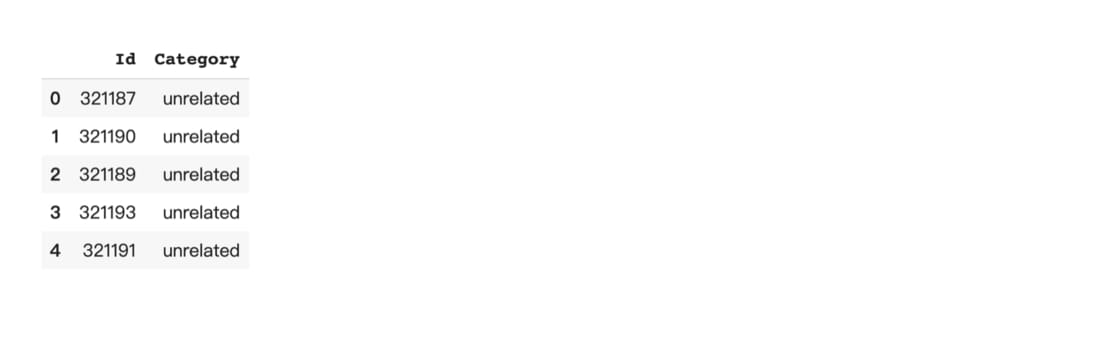!ls bert*.csv

bert_1_prec_training_samples.csv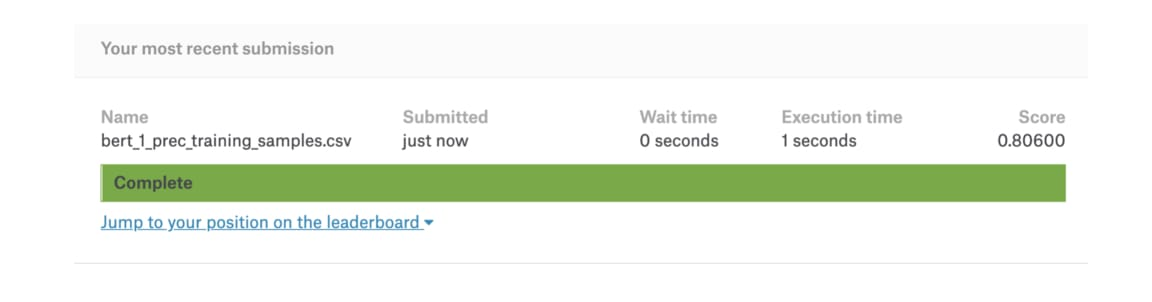predictions = get_predictions(model, trainloader)
df = pd.DataFrame({"predicted": predictions.tolist()})
df['predicted'] = df.predicted.apply(lambda x: index_map[x])
df1 = pd.concat([trainset.df, df.loc[:, 'predicted']], axis=1)
disagreed_tp = ((df1.label == 'disagreed') & \
(df1.label == df1.predicted) & \
(df1.text_a.apply(lambda x: True if len(x) < 10 else False)))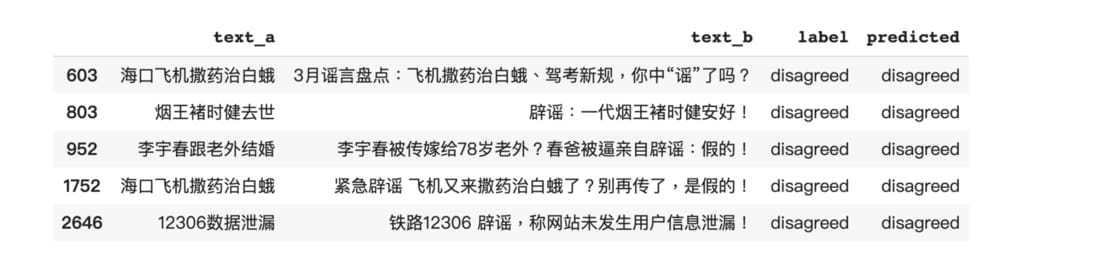# 觀察訓練過後的 model 在處理假新聞分類任務時關注的位置
# 去掉 state_dict 即可觀看原始 BERT 結果
model_version = 'bert-base-chinese'
finetuned_model  = BertModel.from_pretrained(model_version,
output_attentions=True, state_dict=model.state_dict())

# 兩個句子
sentence_a = "烟王褚时健去世"
sentence_b = "辟谣：一代烟王褚时健安好！"

# 得到 tokens 後丟入 BERT 取得 attention
inputs = tokenizer.encode_plus(sentence_a, sentence_b, return_tensors='pt', add_special_tokens=True)
token_type_ids = inputs['token_type_ids']
input_ids = inputs['input_ids']
attention = finetuned_model(input_ids, token_type_ids=token_type_ids)[-1]
input_id_list = input_ids.tolist() # Batch index 0
tokens = tokenizer.convert_ids_to_tokens(input_id_list)
call_html()

# 這段程式碼會顯示下圖中右邊的結果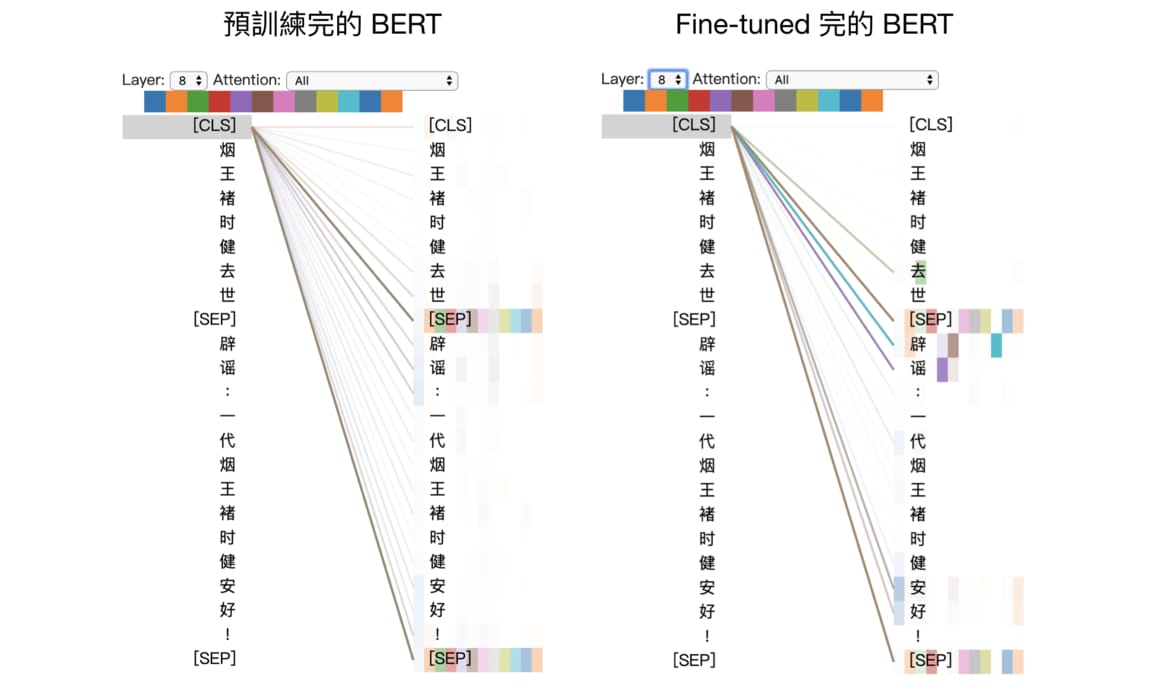• 闢謠
• 去世
• 安好

# 腳本模式的好處是可以透過改變參數快速進行各種實驗。
# 壞處是黑盒子效應，不過對閱讀完本文的你應該不是個問題。
# 選擇適合自己的方式 fine-tuning BERT 吧！

--bert_model bert-base-uncased \
--do_train \
--do_predict \
--do_lower_case \
--train_file $SQUAD_DIR/train-v1.1.json \ --predict_file$SQUAD_DIR/dev-v1.1.json \
--train_batch_size 12 \
--learning_rate 3e-5 \
--num_train_epochs 2.0 \
--max_seq_length 384 \
--doc_stride 128 \


## 結語¶

• 直觀理解 BERT 內部自注意力機制的物理意義
• 向其他人清楚解釋何謂 BERT 以及其運作的原理
• 了解 contextual word repr. 及兩階段遷移學習
• 將文本數據轉換成 BERT 相容的輸入格式
• 依據下游任務 fine tuning BERT 並進行推論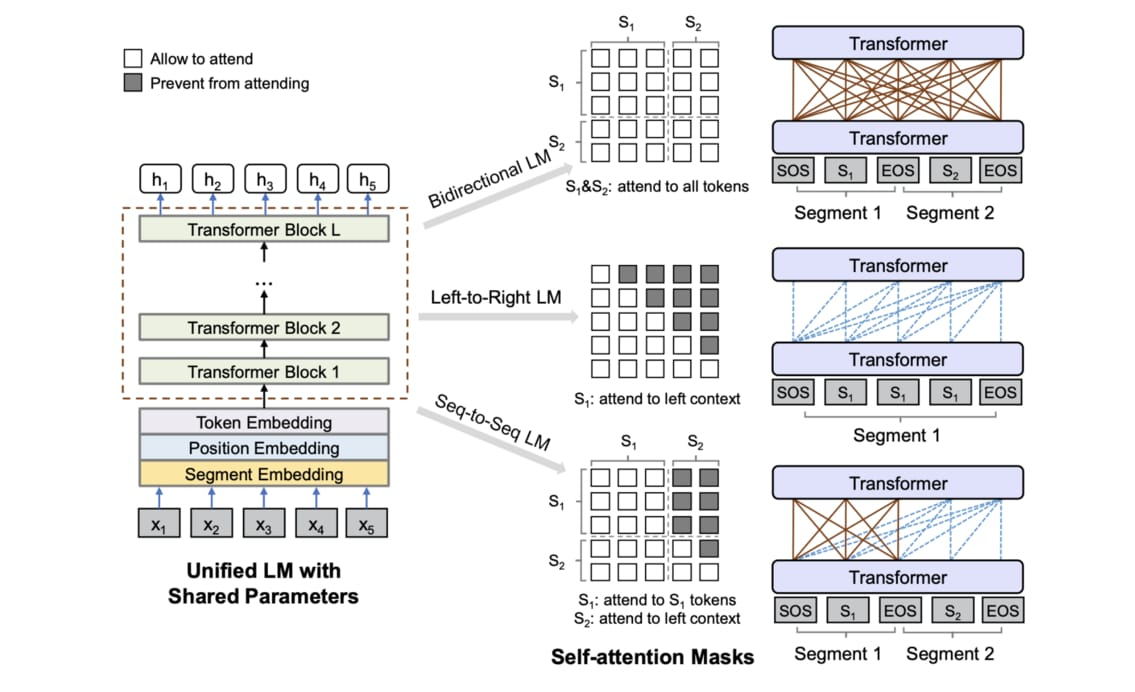UniLM 用 3 種語言模型作為預訓練目標，可以 fine tune 自然語言生成任務，是值得期待的研究 （圖片來源

• BERT 的 Encoder 架構很適合做自然語言理解 NLU 任務，但如文章摘要等自然語言生成 NLG 的任務就不太 okay。BertSum 則是一篇利用 BERT 做萃取式摘要並在 CNN/Dailymail 取得 SOTA 的研究，適合想要在 BERT 之上開發自己模型的人參考作法
• UniLM 透過「玩弄」注意力遮罩使得其可以在預訓練階段同時訓練 3 種語言模型，讓 fine tune NLG 任務不再是夢想。如果你了解之前 Transformer 文章裡說明的遮罩概念，幾秒鐘就能直觀理解上面的 UniLM 架構
• 最近新的 NLP 王者非 XLNet 莫屬。其表現打敗 BERT 自然不需多言，但訓練該模型所需的花費令人不禁思考這樣的大公司遊戲是否就是我們要的未來
• 有些人認為 BERT 不夠通用，因為 Fine-tuning 時還要依照不同下游任務加入新的 Linear Classifier。有些人提倡使用 Multitask Learning 想辦法弄出更通用的模型，而 decaNLP 是一個知名例子。
• PyTorch 的 BERT 雖然使用上十分直覺，如果沒有強大的 GPU 還是很難在實務上使用。你可以嘗試特徵擷取或是 freeze BERT。另外如果你是以個人身份進行研究，但又希望能最小化成本並加快模型訓練效率，我會推薦花點時間學會在 Colab 上使用 TensorFlow Hub 及 TPU 訓練模型